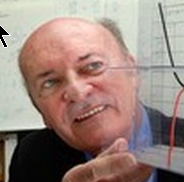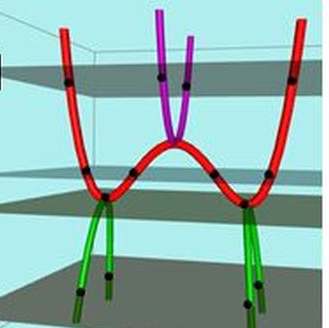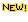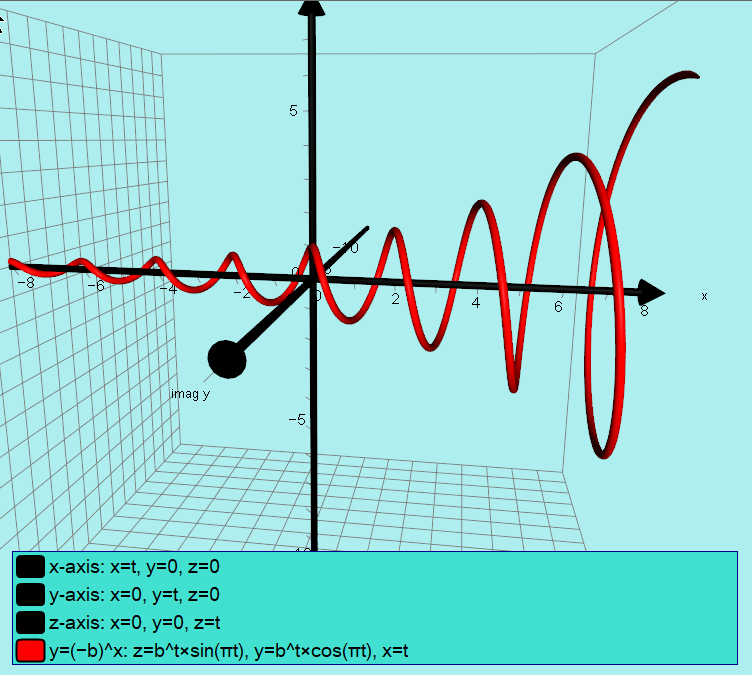# of Philip Lloyd (Aukland NZ)TSM Resources

BlogThe three phantoms of a quartic

Cubic phantoms
Quartic phantoms
Autograph file (.agg)Autograph file (.agg)

y = x^n

y = x^x

The graph of y=x^x (.pdf)
The Spiral Equation (.agg)

y = (–1)^x

Discussion
Autograph file (.agg)

y = (–2)^x

Discussion
Autograph file
(.agg)

y = (–b)^x

Autograph file (.agg)3D visualization of y = (-1.25)^x

y^x = x^y

y = ln(x)

With Complex y-plane (.pdf)

x^6 = x^3

Mapping Phantom Graphs